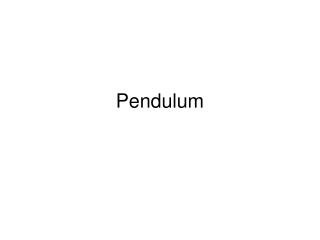DownloadDownload PresentationPendulum

# Pendulum

Télécharger la présentation## Pendulum

- - - - - - - - - - - - - - - - - - - - - - - - - - - E N D - - - - - - - - - - - - - - - - - - - - - - - - - - -
##### Presentation Transcript

1. Pendulum

2. Restoring force: F = -mg sinθ If θ is small, sin θ ≈ θ F = -mg θ = -mg x/L F = -(mg/L) x F = -kx Force constant (k) = mg/L

3. For small angles, pendulum is SHM period: T = 2П √(m/k) = 2 П √(m/(mg/L)) T = 2 П √(L/g) f = (1/ 2 П) √(g/L)

4. k If the mass on the spring and the pendulum have the same period, what is the length of the pendulum? m1g/k

5. Energy of a Pendulum θ L PEmax? vmax?

6. k v M Arrow sticks into block. Maximum compression of spring?